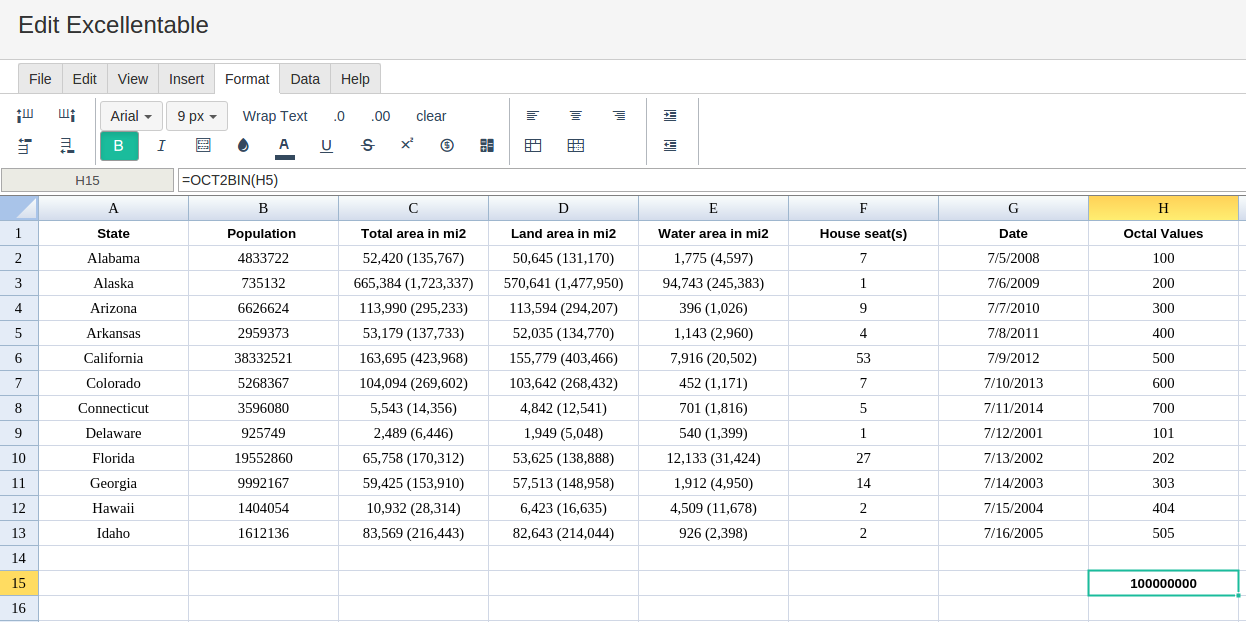### Definition of OCT2BIN

Converts a signed octal number to signed binary format.

### Sample Usage

`OCT2BIN(37,8)`

`OCT2BIN(A2)`

### Syntax

`OCT2BIN(signed_octal_number, [significant_digits])`

• `xsigned_octal_number` - The signed 30-bit octal value to be converted to signed binary, provided as a string.

• The most significant bit of `signed_octal_number` is the sign bit; that is, negative numbers are represented in two's complement format.

• For this function, this value has a maximum of 777 if positive, and a minimum of 7777777000 if negative.

• If `signed_octal_number` is provided as a valid octal number, it will automatically be converted to the appropriate string input. For example, `OCT2BIN(177)` and `OCT2BIN("177")` yield the same result:`1111111`.

• `significant_digits` - [ OPTIONAL ] The number of significant digits to ensure in the result.

• If this is greater than the number of significant digits in the result, the result is left-padded with zeros until the total number of digits reaches `significant_digits`.

• This value is ignored if the most significant bit of `signed_octal_number` is `1`; that is, if the expressed `signed_octal_number` is greater than or equal to 4000000000.

### Notes

• As with any octal value, only the digits 0-7 are valid. Digits outside of this will cause `OCT2BIN` to return a `#NUM!` error.

• If the number of digits required is greater than the specified `significant_digits`, the `#NUM!` error is returned.

`OCT2HEX`: Converts a signed octal number to signed hexadecimal format.

`OCT2DEC`: Converts a signed octal number to decimal format.

`HEX2OCT`: Converts a signed hexadecimal number to signed octal format.

`HEX2DEC`: Converts a signed hexadecimal number to decimal format.

`HEX2BIN`: Converts a signed hexadecimal number to signed binary format.

`DEC2OCT`: Converts a decimal number to signed octal format.

`DEC2HEX`: Converts a decimal number to signed hexadecimal format.

`DEC2BIN`: Converts a decimal number to signed binary format.

`BIN2OCT`: Converts a signed binary number to signed octal format.

`BIN2HEX`: Converts a signed binary number to signed hexadecimal format.

`BIN2DEC`: Converts a signed binary number to decimal format.

###Error.

User does not have sufficient privileges to access this Content